# Convert Pace to MPH and Back Again in Excel

Excel is a great tool for figuring stuff out, like for conversions that aren’t easy to do in your head. Here I’m converting Pace to MPH and then reversing the process, converting MPH to Pace, to create a conversion chart.

## My Conversion Problem for Minutes per Mile to MPH

I track my Average Pace when out walking for exercise by using the iPhone App Walkmeter, then log that information into the Lose It App. The problem I have is converting my Average Pace to Miles per Hour (MPH).

Below you can see my average pace is 13:22 per mile, but Lose It wants me to pick from a list of MPH values.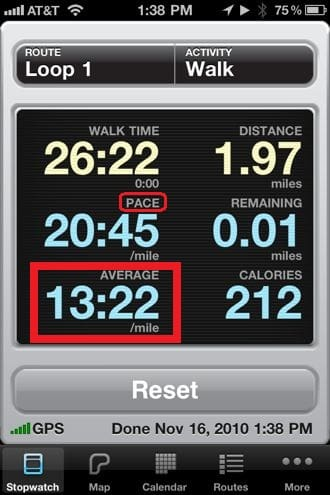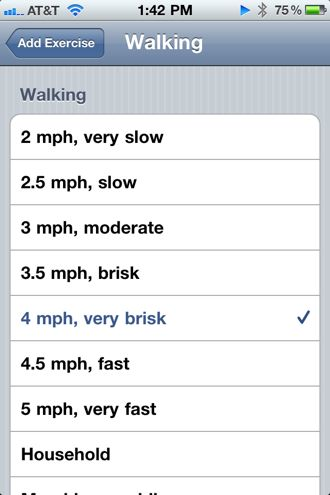## Simple Conversion Equation

You can use algebra to work out how to convert Pace, in minutes per mile, to MPH.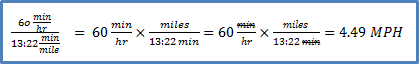The problem with this is that Walkmeter shows the Average Pace in Minutes and Seconds per mile, which is not decimal minutes per mile.

## Converting Minutes and Seconds

There are a couple of ways to convert minutes:seconds to decimal minutes. The first mimics what I would do by using a calculator and the second is strictly an Excel thing to convert minutes per mile to MPH.

### Decimal Minutes

Divide the seconds by 60 then add the result to the number of minutes to get decimal minutes.

B2 =MINUTE(A2)+SECOND(A2)/60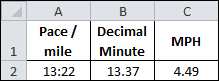This solution uses the Excel Functions MINUTE and SECOND.

### Decimal Hours

Convert 13:22 to a time serial number by using the TIME Function, then multiply by 24 to get decimal hours.

B2 =TIME(,MINUTE(A2),SECOND(A2))*24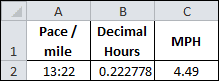The TIME Function above has three arguments:

• Hour, which is blank
• Minute, which uses the MINUTE Function
• Second, which uses the SECOND Function

## Convert to MPH

Now that I’ve converted minutes and seconds to either decimal minutes or hours, converting Pace to MPH can be completed in a second step.

### Using Decimal Minutes

Simply divide 60 min/hr by the 13.37 pace/mile to get 4.49 MPH.

C2 =60/B2Combining equations in cells B2 and C2 gets us the conversion in one big equation:

MPH =60/(MINUTE(A2)+SECOND(A2)/60)

### Using Decimal Hours

Here we simply invert the decimal hours to get our answer, one (1) divided by 0.0222778 decimal hours, gives us 4.49 MPH.

C2 =1/B2Again, we can combine equations to get:

MPH =1/(TIME(,MINUTE(A2),SECOND(A2))*24)

## MPH to Pace Conversion

For a given set of MPH values I want to convert to Pace per mile and show the result in a minutes:seconds format. Essentially reversing what I just did above.

The simplest way to do this is to realize that the time serial number is based on seconds. We’ll also use the fact that 1 hour = 3600 seconds.

When we divide 3600 by an MPH value, it gives us the number of seconds it takes to go one mile. Plugging these seconds into the TIME Function will give us our answer, but as a time serial number. We can then use a custom format of mm:ss for the Pace range and the conversion is complete. The equation for cell B2 is:

=TIME(,,3600/A2)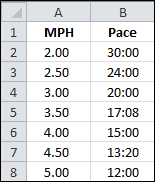This formula works because column B is formatted using the mm:ss custom format.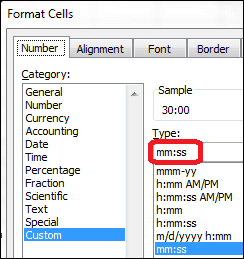So now I have a conversion chart for MPH to Pace and can probably remember that a pace of 13:22 is close to 4.5 MPH, which is helpful to me. How about you?## 9 thoughts on “Convert Pace to MPH and Back Again in Excel”

1. Pingback: Aircraft Models and Kits » Blog Archive » Jet Model Airplanes Video

2.David Kravitz

I’ve followed your advice to convert mph to pace, but my attempts to convert pace to mph have failed. I suspect it has to do with the formatting of the cell in which I enter the pace. I’ve tried the custom mm:ss as used for the speed to pace conversion, but that doesn’t seem to work. Suggestions? Thanks – and thanks for this posting.

1.Gregory

The formatting for MPH is either General or Number, which shouldn’t be the problem. Assuming the MPH value is in cell B2 the formula

=60/(MINUTE(B2)+SECOND(B2)/60)

will convert to Pace, as will the formula

=1/(TIME(,MINUTE(B2),SECOND(B2))*24)

Just copy either formula directly from this comment and a paste it into the formula bar of your Excel worksheet. You can change the B2 cell references if your MPH value is in another cell.

3.Jeff

To respond to David’s question from above, was able to get 13:22 to display correctly in A2 and get the correct values in B2 and C2 by entering the following in A2 in conjunction with mm:ss formatting…

=((13*60)+22)/86400

86400 being the number of seconds in a day.

4.JoBier

Trying to compute an average of paces doesn’t work. I assume its because Excel is treating these as times (time of day) and not timespans.

Ideas?

1.Gregory

The average of paces is really and average of averages. The problem is that every pace value in a list is “per mile” and disregards how many miles. To get a weighted average for pace you have to sum the miles, then sum the minutes, then divide the sum of minutes by the sum of miles.

An example with two data points:
1) 10 miles in 60 minutes, a pace of 6 minutes per mile, and
2) 1 mile in 30 minutes, a pace of 30 minutes per mile.

The average of averages is (30 + 6) / 2 = 18 minutes per mile, and ignores the number of miles at a particular pace.

The true pace or weighted average is (60 + 30) / (10 + 1) = 8.18 minutes per mile.

5.JoBier

So guess my question is more general then, how do you get Excel to compute and *display* an average of timespans?

1.Gregory

I can use =AVERAGE(B2:B8) to get the answer 18:47 but have to have the same cell formatting as the pace range.

6.JoBier

Odd, tried again with the same formatting and it still didn’t work.